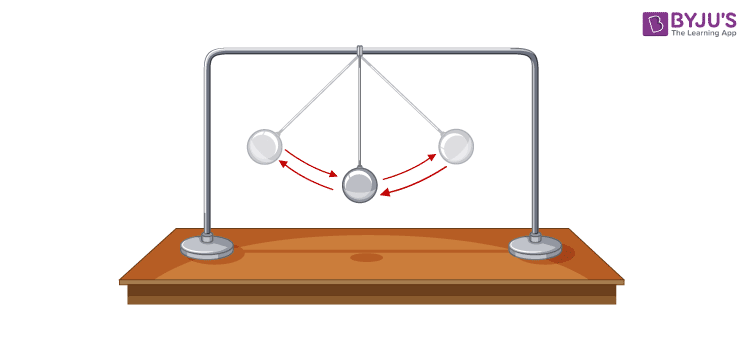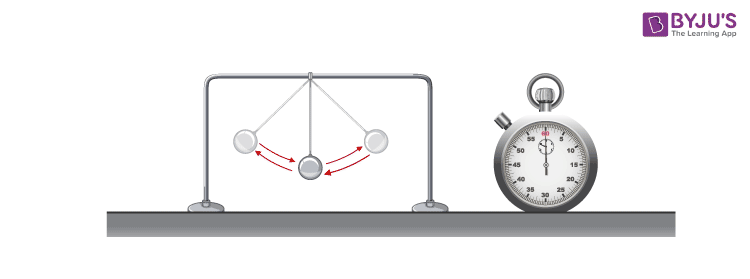Jet Set Go! All about Aeroplanes Jet Set Go! All about Aeroplanes

# Changing the Period of a PendulumA pendulum consists of a mass at the end of a thin cord, known as the bob. It is connected at a fixed point. When the mass is drawn towards high and left, the gravitational force accelerates it back to its previous position. Changing the length of the pendulum changes its period. Long-length pendulums swing with a smaller frequency and therefore have a longer period. The mass on a pendulum doesn’t affect the swing of the pendulum. Now you might wonder why mass does not affect a pendulum’s period. This is because the period of a pendulum is only affected by the length of the string and the acceleration due to gravity. The formula for the time period of a simple pendulum is given by the equation

$$\begin{array}{l}T=2\pi \sqrt{\frac{L}{g}}\end{array}$$
where L is the length of the string and g is the force of gravity.

## Pendulum Swing Experiment

In the pendulum swing experiment given here, you will explore the factors that affect the speed and duration of a pendulum’s swing, which is also known as an oscillating motion. Given below is a detailed and methodical experiment with a concise conclusion.

### Aim:

To change the period of a pendulum

### Apparatus Required:• Weights
• Stopwatch
• Tape
• Ruler
• Straw
• Table

### Procedure

• Tie a weight to a thread.
• Tie the thread to the straw and tape the straw on the table in such a way that around half of an inch hangs over the edge.
• Tape the other end of the thread with the table in such a manner that the length from the straw’s end to the middle of the weight is 4 inches.
• Let the pendulum settle and be constant.
• Pull the bob about one inch and leave it gently. Make sure to make the pendulum swing in a steady arc.
• When you let it go, start the stopwatch and note the number of times it swings from its initial point and back to the original until you count at least 10. Repeat this three times and take the average collected data from the three trials. The no of swings divided by the time taken is called the period of the pendulum.
• Extend the length of the pendulum to 5 inches and repeat the procedure.
• Increase the weight of the bobs and repeat the above procedure.

### Conclusion

Only the length affects the period of a pendulum. Changing the weights and the distance pulled to swing does not affect the time taken to finish a swing from an initial to the final position. The period of the pendulum remains the same in both cases.

## Frequently Asked Questions – FAQs

### What is a simple pendulum?

A simple pendulum is a point mass suspended by a weightless, inextensible string fixed rigidly to support.

### What is an expression for the time period of a simple pendulum?

T = 2π√(l/g)
Where l is the length of the pendulum.
g is the acceleration due to gravity.

### When will the motion of a simple pendulum be simple harmonic motion?

The motion of a simple pendulum will be simple harmonic motion if its angular displacement θ is very small.

### When a pendulum is taken on top of the mountain, will the time period increase or decrease?

The time period increases as ‘g’ decreases, so it gains time.

### Why a simple pendulum does not vibrate at the centre of the earth?

At the centre of the earth, g = 0
Therefore, T = 2π√(l/g) = ∞
The pendulum will take infinite time to complete one vibration. In other words, the pendulum will not vibrate at the centre of the earth

The project mentioned above would have shown the bright and interactive side of science, which is not yet noticed by people but is highly beneficial for students in particular. Implementing such projects in schools and doing them at home would make children interested and fascinated in knowing about science subjects better over the years. Stay tuned to BYJU’S to learn more innovative project ideas.

## Watch the video to understand the concept of simple harmonic motion in detail.Test Your Knowledge On Changing The Period Of A Pendulum!